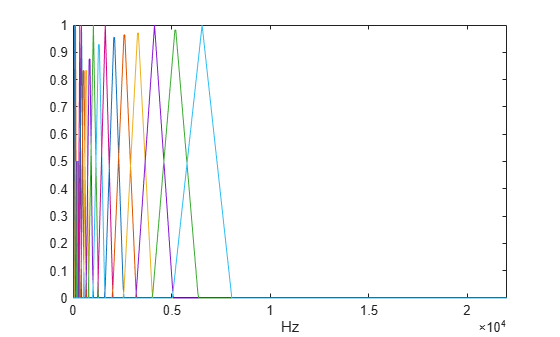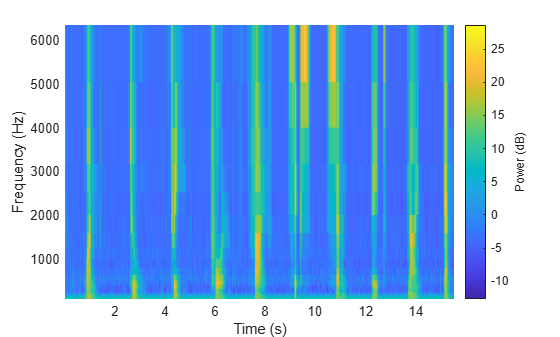# cepstralCoefficients

Extract cepstral coefficients

## Syntax

``coeffs = cepstralCoefficients(S)``
``coeffs = cepstralCoefficients(S,Name,Value)``

## Description

example

````coeffs = cepstralCoefficients(S)` returns the cepstral coefficients over time. The input, `S`, must be a real-valued spectrogram or auditory spectrogram.```

example

````coeffs = cepstralCoefficients(S,Name,Value)` specifies options using one or more `Name,Value` pair arguments.For example, ```coeffs = cepstralCoefficients(S,'Rectification','cubic-root')``` uses cubic-root rectification to calculate the coefficients.```

## Examples

collapse all

Read an audio file into the workspace.

`[audioIn,fs] = audioread('SpeechDFT-16-8-mono-5secs.wav');`

Convert the audio signal to a frequency-domain representation using 30 ms windows with 15 ms overlap. Because the input is real and therefore the spectrum is symmetric, you can use just one side of the frequency domain representation without any loss of information. Convert the complex spectrum to the magnitude spectrum: phase information is discarded when calculating mel frequency cepstral coefficients (MFCC).

```windowLength = round(0.03*fs); overlapLength = round(0.015*fs); S = stft(audioIn,"Window",hann(windowLength,"periodic"),"OverlapLength",overlapLength,"FrequencyRange","onesided"); S = abs(S);```

Design a one-sided frequency-domain mel filter bank. Apply the filter bank to the frequency-domain representation to create a mel spectrogram.

```filterBank = designAuditoryFilterBank(fs,'FFTLength',windowLength); melSpec = filterBank*S;```

Call `cepstralCofficients` with the mel spectrogram to create MFCC.

`melcc = cepstralCoefficients(melSpec);`

Read an audio signal and convert it to a one-sided magnitude short-time Fourier transform. Use a 50 ms periodic Hamming window with a 10 ms hop.

```[audioIn,fs] = audioread('NoisySpeech-16-22p5-mono-5secs.wav'); windowLength = round(0.05*fs); hopLength = round(0.01*fs); overlapLength = windowLength - hopLength; S = stft(audioIn,"Window",hamming(windowLength,'periodic'),"OverlapLength",overlapLength,"FrequencyRange","onesided"); S = abs(S);```

Design a one-sided frequency-domain gammatone filter bank. Apply the filter bank to the frequency-domain representation to create a gammatone spectrogram.

```filterBank = designAuditoryFilterBank(fs,'FFTLength',windowLength,"FrequencyScale","erb"); gammaSpec = filterBank*S;```

Call `cepstralCoefficients` with the gammatone spectrogram to create gammatone frequency cepstral coefficients. Use a cubic-root rectification.

`gammacc = cepstralCoefficients(gammaSpec,"Rectification","cubic-root");`

Cepstral coefficients are commonly used as compact representations of audio signals. Generally, they are calculated after an audio signal is passed through a filter bank and the energy in the individual filters is summed. Researchers have proposed various filter banks based on psychoacoustic experiments (such as mel, Bark, and ERB). Using the `cepstralCoefficients` function, you can define your own custom filter bank and then analyze the resulting cepstral coefficients.

Read in an audio file for analysis.

`[audioIn,fs] = audioread('Counting-16-44p1-mono-15secs.wav');`

Design a filter bank that consists of 20 triangular filters with band edges over the range 62.5 Hz to 8000 Hz. Spread the filters evenly in the log domain. For simplicity, design the filters in bins. Most popular auditory filter banks are designed in a continuous domain, such as Hz, mel, or Bark, and then warped back to bins.

```numFilters =20; filterbankStart =62.5; filterbankEnd =8000; numBandEdges = numFilters + 2; NFFT = 1024; filterBank = zeros(numFilters,NFFT); bandEdges = logspace(log10(filterbankStart),log10(filterbankEnd),numBandEdges); bandEdgesBins = round((bandEdges/fs)*NFFT) + 1; for ii = 1:numFilters filt = triang(bandEdgesBins(ii+2)-bandEdgesBins(ii)); leftPad = bandEdgesBins(ii); rightPad = NFFT - numel(filt) - leftPad; filterBank(ii,:) = [zeros(1,leftPad),filt',zeros(1,rightPad)]; end```

Plot the filter bank.

```frequencyVector = (fs/NFFT)*(0:NFFT-1); plot(frequencyVector,filterBank'); xlabel('Hz') axis([0 frequencyVector(NFFT/2) 0 1])```Transform the audio signal using the `stft` function, and then apply the custom filter bank. Apply the filter bank to the frequency-domain representation to create a custom auditory spectrogram. Plot the spectrogram.

```[S,~,t] = stft(audioIn,fs,"Window",hann(NFFT,'periodic'),"FrequencyRange","twosided"); S = abs(S); spec = filterBank*S; surf(t,bandEdges(2:end-1),10*log10(spec),'EdgeColor','none') view([0,90]) axis([t(1) t(end) bandEdges(2) bandEdges(end-1)]) xlabel('Time (s)') ylabel('Frequency (Hz)') c = colorbar; c.Label.String = 'Power (dB)';```Call `cepstralCoefficients` with the custom auditory spectrogram to create custom cepstral coefficients.

`ccc = cepstralCoefficients(S);`

Create a `dsp.AudioFileReader` object to read in audio frame-by-frame. Create a `dsp.AsyncBuffer` object to buffer the input into overlapped frames.

```fileReader = dsp.AudioFileReader("Ambiance-16-44p1-mono-12secs.wav"); buff = dsp.AsyncBuffer;```

Design a two-sided mel filter bank that is compatible with 30 ms windows.

```windowLength = round(0.03*fileReader.SampleRate); filterBank = designAuditoryFilterBank(fileReader.SampleRate,"FFTLength",windowLength,"OneSided",false);```

In an audio stream loop:

1. Read a frame of data from the audio file.

2. Write the frame of data to the buffer.

3. If enough data is available for a hop, read a 30 ms frame of data from the buffer with a 20 ms overlap between frames.

4. Transform the data to a magnitude spectrum.

5. Apply the mel filter bank to create a mel spectrum.

6. Call `cepstralCoefficients` to return the mel frequency cepstral coefficients (MFCC).

```win = hann(windowLength,'periodic'); overlapLength = round(0.02*fileReader.SampleRate); hopLength = windowLength - overlapLength; while ~isDone(fileReader) audioIn = fileReader(); write(buff,audioIn); while buff.NumUnreadSamples > hopLength x = read(buff,windowLength,overlapLength); X = abs(fft(x.*win)); melSpectrum = filterBank*X; melcc = cepstralCoefficients(melSpectrum); end end```

## Input Arguments

collapse all

Spectrogram or auditory spectrogram, specified as an L-by-M matrix or L-by-M-by-N 3-D array, where:

• L –– Number of frequency bands

• M –– Number of frames

• N –– Number of channels

Data Types: `single` | `double`

### Name-Value Pair Arguments

Specify optional comma-separated pairs of `Name,Value` arguments. `Name` is the argument name and `Value` is the corresponding value. `Name` must appear inside quotes. You can specify several name and value pair arguments in any order as `Name1,Value1,...,NameN,ValueN`.

Example: `'NumCoeffs',16`

Number of coefficients returned for each window of data, specified as the comma-separated pair consisting of `'NumCoeffs'` and a positive integer greater than one.

Data Types: `single` | `double` | `int8` | `int16` | `int32` | `int64` | `uint8` | `uint16` | `uint32` | `uint64`

Type of nonlinear rectification applied prior to the discrete cosine transform, specified as the comma-separated pair consisting of `'Rectification'` and `'log'` or `'cubic-root'`.

Data Types: `char` | `string`

## Output Arguments

collapse all

Cepstral coefficients, returned as an M-by-B matrix or M-by-B-by-N array, where:

• M –– Number of frames (columns) of the input.

• B –– Number of coefficients returned per frame. This is determined by `NumCoeffs`.

• N –– Number of channels (pages) of the input.

Data Types: `single` | `double`

## Algorithms

Given a time-frequency representation, the `cepstralCoefficients` function performs the following operations on each spectrum, individually, as described in :

1. Rectifies the spectrum by applying a logarithm or cubic-root, as specified by the `Rectification` parameter.

2. Transforms the rectified spectrum using the DCT-II transform.

3. Returns the first `NumCoeffs` from the cepstral representation.

 Rabiner, Lawrence R., and Ronald W. Schafer. Theory and Applications of Digital Speech Processing. Upper Saddle River, NJ: Pearson, 2010.

## SupportGet trial now